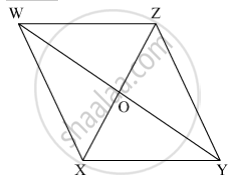SSC (English Medium) Class 8Maharashtra State Board
Share

# Referring the Adjacent Figure of a Parallelogram, Write the Answer of Questions Given Below. - SSC (English Medium) Class 8 - Mathematics

ConceptKinds of Quadrilaterals Concept of Parallelogram

#### Question

Referring the adjacent figure of a parallelogram, write the answer of questions given below.(1) If l(WZ) = 4.5 cm then l(XY) = ?
(2) If l(YZ) = 8.2 cm then l(XW) = ?
(3) If l(OX) = 2.5 cm then l(OZ) = ?
(4) If l(WO) = 3.3 cm then l(WY) = ?
(5) If m∠WZY = 120° then m∠WXY = ? and m∠XWZ = ?

#### Solution

WXYZ is a parallelogram.

(1)
l(XY) = l(WZ) = 4.5 cm                 (Opposite sides of a parallelogram are congruent)

(2)
l(XW) = l(YZ) = 8.2 cm                 (Opposite sides of a parallelogram are congruent)

(3)
l(OZ) = l(OX) = 2.5 cm                  (Diagonals of parallelogram bisect each other)

(4)
l(WY) = 2 × l(WO) = 2 × 3.3 = 6.6 cm         (Diagonals of parallelogram bisect each other)

(5)
m∠WXY = m∠WZY = 120º      (Opposite angles of a parallelogram are congruent)

Now,

m∠WZY + m∠XWZ = 180º      (Adjacent angles of a parallelogram are supplementary)

⇒ 120º + m∠XWZ = 180º

⇒ m∠XWZ = 180º − 120º = 60º

Is there an error in this question or solution?

#### APPEARS IN

Balbharati Solution for Balbharati Class 8 Mathematics (2019 to Current)
Chapter 8: Quadrilateral : Constructions and Types
Practice Set 8.3 | Q: 2 | Page no. 50
Solution Referring the Adjacent Figure of a Parallelogram, Write the Answer of Questions Given Below. Concept: Kinds of Quadrilaterals - Concept of Parallelogram.
S